GeeksforGeeks App
Open AppBrowser
Continue

# Deep Dive into Error Handling in JavaScript/Node.js

What is an Error?

An error is an illegal operation performed by the user which results in the abnormal working of the program.

Types of Errors: Errors can be broadly categorized as

• Operational Errors: Errors that happen while a program is undertaking a task. For instance, network failure would be an operational error
• Developer Errors: This type of error occurs when a developer has made a mistake. The main example of this is invalid input. In these cases, the program should not attempt to continue running and should instead crash with a helpful description so that the developer can address their mistake

Throwing Errors: Typically an input error can be dealt with the use of the throw keyword.

Example:

## Javascript

 `function` `doTask(amount) {``    ``if` `(``typeof` `amount !== ``'number'``) ``        ``throw` `new` `Error(``'amount must be a number'``)``    ``return` `amount / 2``}`` ` `doTask(``"This will generate an error"``)`

Output: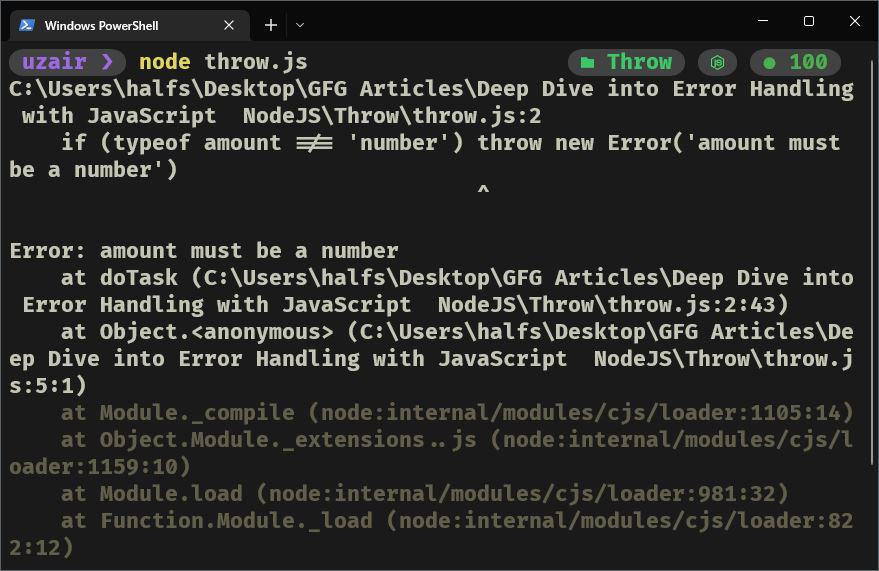As we can observe that when the program is run, we get an Error stating that the amount must be a number.

Thus we can restrict the types of inputs that can be accepted by our program and then stop the execution of the program so that no unexpected or no wrong output is generated.

Native Error Classes in JavaScript: There are six other native error constructors that inherit from the base Error constructor, these are:

• ReferenceError
• EvalError
• SyntaxError
• RangeError
• TypeError
• URIError

These error constructors exist mostly for native JavaScript API and functionality.

1. Reference Error: When we use a variable that is not defined or which is yet to be defined the Error of type ReferenceError is thrown

Syntax:

`node -p "thisVariableIsNotDefined"`

Example:

## Javascript

 `const defined = ``"This variable is defined"``;``console.log(defined);``console.log(notDefined); ``// There is no such declaration for ``// a variable named notDefined and ``// thus results in a reference error`

Output: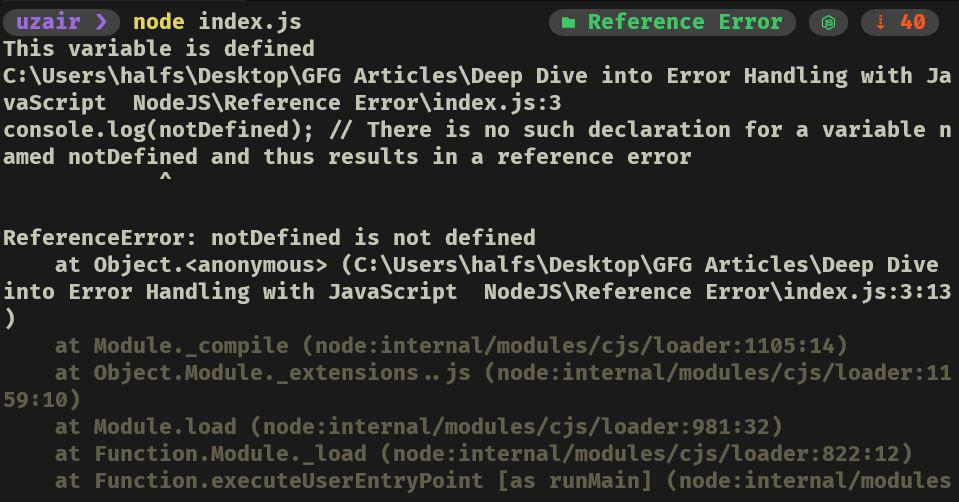This is because there is no variable named thisVariableIsNotDefined, and thus, a ReferenceError is thrown.

2. EvalError: The EvalError object indicates an error regarding the global eval() function. This exception is not thrown by JavaScript anymore. However, the EvalError object remains for compatibility.

Since the Error isn’t thrown by javascript anymore, we can manually throw the EvalError object.

Syntax:

```throw new EvalError('message',
'file_where_error_occurs', 'line_number')```

Example: A very popular example of EvalError is where we try to divide a number by 0, below is the code snippet which emulates the same, now note that by default the JavaScript engine does not throw EvalError so we need to manually throw an error. Take a look at the example below and then we shall discuss what exactly is happening in the code snippet.

## Javascript

 `try` `{``    ``const a = 7;``    ``const b = 0;``    ``if` `(b === 0) ``        ``throw` `new` `EvalError(``"Cannot divide a number by 0"``);``    ``let quot = eval(a / b);``    ``console.log(`Quotient = \${quot}`);``} ``catch` `(err) {``    ``console.log(``"Error is of Type : "` `+ err.name);``    ``console.log(``"Error Message : "` `+ err.message);``}`

Output: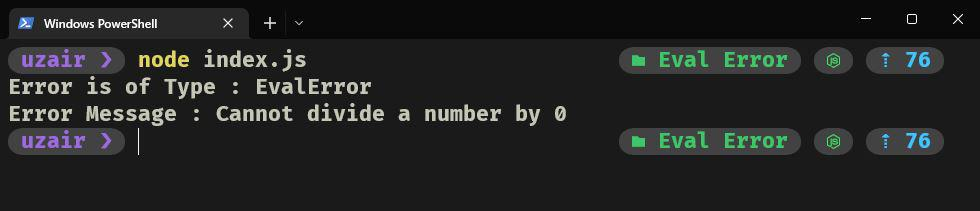We declare two variables namely a and b and initialize them with some numeric value. Now we check if variable b is equal to 0 and if so throw a new EvalError and then handle it in the try/catch block, where we print the error type and the error message.

3. SyntaxError: The eval() function evaluates JavaScript code represented as a string and returns its completion value.

To understand how eval() consider the example below: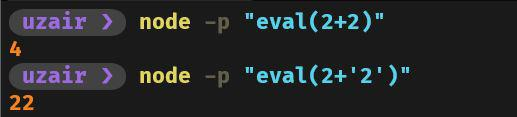As you can see that an operator, alongside two operands, is required by the eval() function.

Let us now see what happens if you do not pass an operator in the eval() function: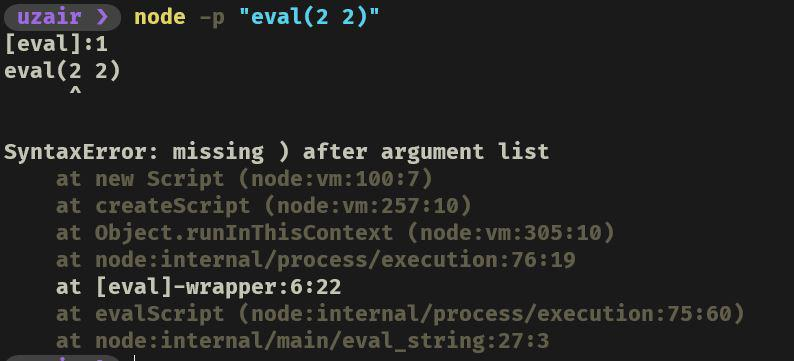We can observe that the SyntaxError occurs as the eval() function requires an operator along with two operands and thus it throws an error; as there is no operand to be found.

4. RangeError: A RangeError is thrown when an out-of-bounds value is encountered. For example, in a bank application where the balance of the account cannot be negative.

## Javascript

 `try` `{``    ``const a = 7;``    ``const b = 0;``    ``if` `(b === 0) ``        ``throw` `new` `EvalError(``"Cannot divide a number by 0"``);``    ``let quot = eval(a / b);``    ``console.log(`Quotient = \${quot}`);``} ``catch` `(err) {``    ``console.log(``"Error is of Type : "` `+ err.name);``    ``console.log(``"Error Message : "` `+ err.message);``}`

Output: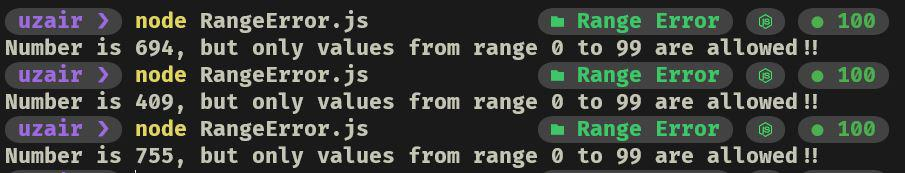The above block of code is very easy to understand, what it does is that it generates a random 3-digit number, but take a look at the if condition. We have specified that only values from 0 to 99 are allowed, now since the random() function generates only 3 digit number, the range error occurs.

5. TypeError: The TypeError object represents an error when an operation could not be performed, typically (but not exclusively) when a value is not of the expected type. Consider the following example where we write a function to add two numbers and expect that both the arguments passed to the function are of type number, if not then throw a TypeError:

## Javascript

 `const num1 = 2;``const num2 = ``"This is a string"``;``const add = (var1, var2) => {``    ``try` `{``        ``if` `(``typeof` `var1 !== ``"number"` `        ``|| ``typeof` `var2 !== ``"number"``)``            ``throw` `new` `TypeError(``                ``"Only numeric values are allowed"``);``        ``return` `var1 + var2;``    ``} ``catch` `(err) {``        ``console.log(err.message);``    ``}``};``add(num1, num2);`

Output: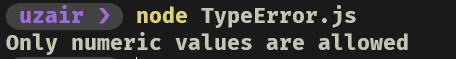The above block of code upon execution will result in TypeError as one of the arguments passed is of type string, which is obviously not a number.

6. URIError: URIError occurs if the encoding or decoding of URI is unsuccessful. This error is thrown as somewhere in the code, the URI encoding or decoding is unsuccessful.

Consider the lines of code in the following snippets:

First is the example of valid URI Encoding: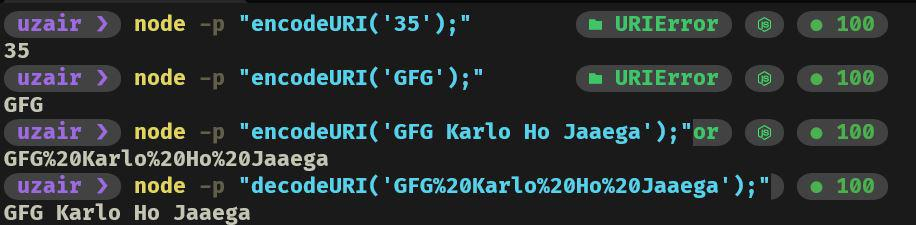Here is another example we pass an invalid encoding string which results in URIError, which outputs the string ‘URI Malformed’: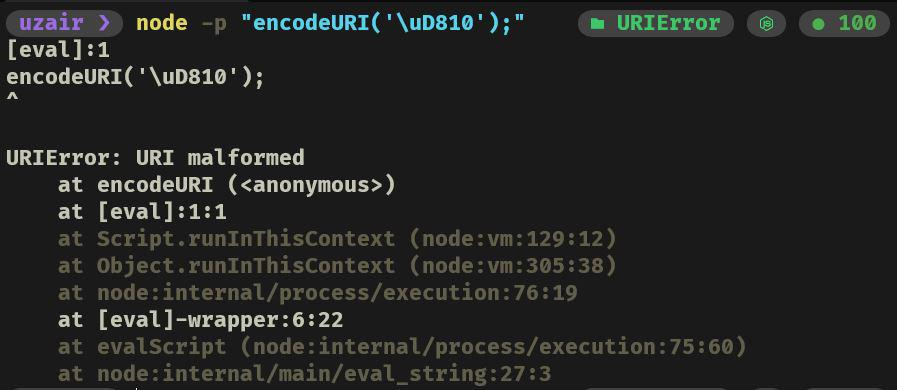We can also verify the error object and check if the error object is an instance of a particular class.

Consider the examples below: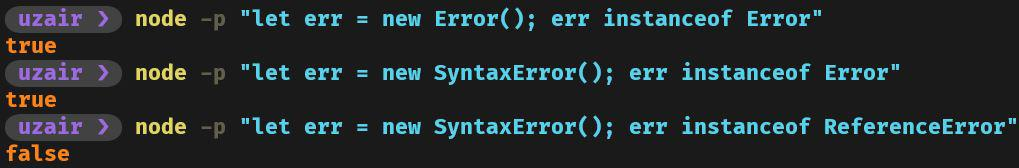First, we check if the Error is an instance of the Error Class that returns true, then we check if SyntaxError is an instance of the Error Class that returns true because the SyntaxError is inherited from the Error class. Lastly, we check if SyntaxError is an instance of ReferenceError Class which returns false because the SyntaxError is inherited from the Error class and not ReferenceError Class.

Custom Errors: Let’s update the doTask  function to throw a custom error when the entered amount is an odd number, here is the implementation of the doTask  function

## Javascript

 `function` `doTask(amount) {``    ``if` `(``typeof` `amount !== ``'number'``) ``        ``throw` `new` `TypeError(``'amount must be a number'``)``    ``if` `(amount <= 0) ``        ``throw` `new` `RangeError(``            ``'amount must be greater than zero'``)``    ``if` `(amount % 2) {``        ``const err = Error(``'amount must be even'``)``        ``err.code = ``'ERR_NUM_MUST_BE_EVEN'``        ``throw` `err``    ``}``    ``return` `amount / 2``}`` ` `doTask(3)`

Upon running the above code snippet this is what we get in the output window: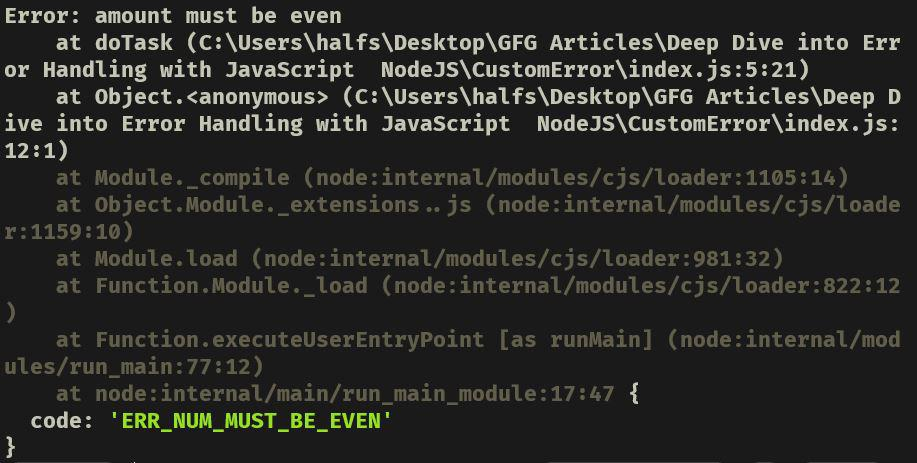Custom Error

This is one way of defining a custom error, another method to create a custom error by inheriting from the Error Class.

Here is an implementation of a custom Error Class.

## Javascript

 `class OddError extends Error {``  ``constructor (varName = ``''``) {``    ``super``(`\${varName} must be even`)``  ``}``  ``get name () { ``return` `'OddError'` `}``}`` ` `function` `doTask (amount) {``  ``if` `(``typeof` `amount !== ``'number'``) ``      ``throw` `new` `TypeError(``'amount must be a number'``)``  ``if` `(amount <= 0) ``      ``throw` `new` `RangeError(``'amount must be greater than zero'``)``  ``if` `(amount % 2) ``throw` `new` `OddError(``'amount'``)``  ``return` `amount / 2``}`` ` `doTask(3)`

Upon executing the above snippet code we get something like this in the output window-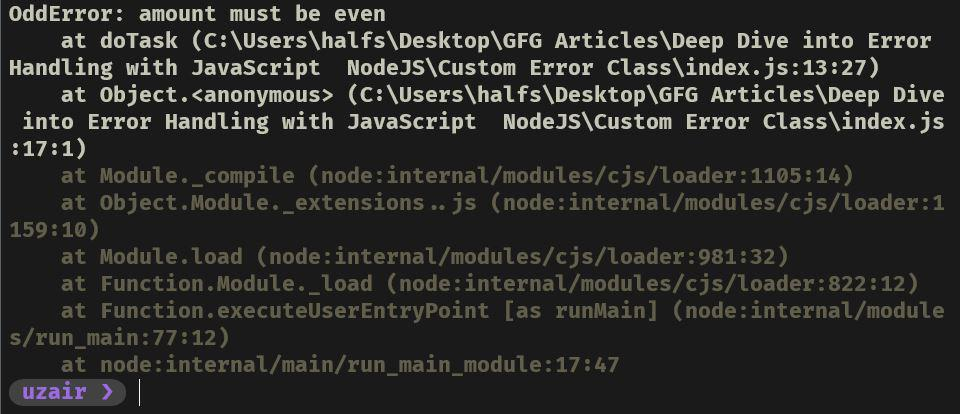Custom Error Class

We can also add the code property to our custom error class; We can make changes to OddError Class and do something like this:

## Javascript

 `class OddError extends Error {``    ``constructor(varName = ``''``) {``        ``super``(varName + ``' must be even'``)``        ``this``.code = ``'ERR_MUST_BE_EVEN'``    ``}``    ``get name() {``        ``return` `'OddError ['` `+ ``this``.code + ``']'``    ``}``}`

When we use the above-updated custom error class we also get the error code in the output when the Error occurs –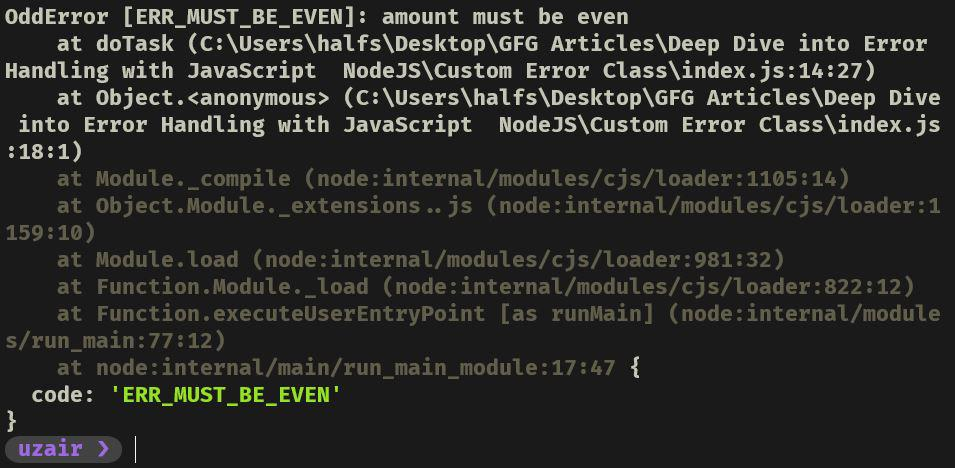Custom Error Class with Error Code

Error Handling Using Try/Catch Block: When an error is thrown in a normal synchronous function it can be handled with a try/catch block.

Using the same code from the previous section, we’ll wrap the doTask(3) function call with a try/catch block:

## Javascript

 `try` `{``    ``const result = doTask(3)``    ``console.log(``'Result : '``, result)``} ``catch` `(err) {``    ``console.error(``'Error caught : '``, err)``}`

Executing this updated code will result in the following: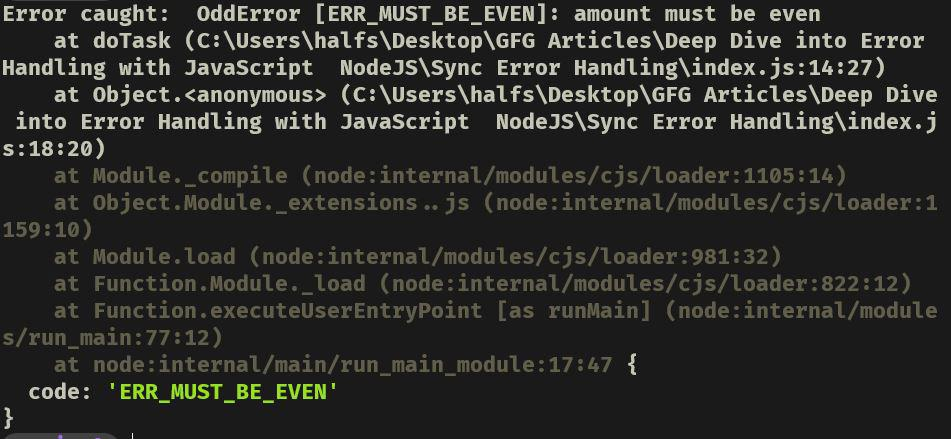In this case, we controlled how the error was output to the terminal but with this pattern, we can also apply any error handling measure as the scenario requires.

Let’s update the argument passed to doTask to a valid input:

## Javascript

 `try` `{``    ``const result = doTask(4)``    ``console.log(``'result'``, result)``} ``catch` `(err) {``    ``console.error(``'Error caught: '``, err)``}`

This will result in the following output: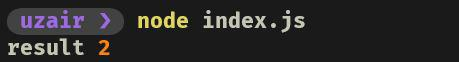Rejection: The throw in a synchronous context is known as an exception. When a promise rejects, it’s representing an asynchronous error. One way to think about exceptions and rejections is that exceptions are synchronous errors and rejections are asynchronous errors.

Let’s imagine that doTask has some asynchronous work to do, so we can use a callback-based API or we can use a promise-based API (even async/await is promise-based).

Let’s convert doTask to return a promise that resolves to a value or rejects if there’s an error:

## Javascript

 `function` `doTask(amount) {``    ``return` `new` `Promise((resolve, reject) => {``        ``if` `(``typeof` `amount !== ``'number'``) {``            ``reject(``new` `TypeError(``'amount must be a number'``))``            ``return``        ``}``        ``if` `(amount <= 0) {``            ``reject(``new` `RangeError(``'amount must be greater than zero'``))``            ``return``        ``}``        ``if` `(amount % 2) {``            ``reject(``new` `OddError(``'amount'``))``            ``return``        ``}``        ``resolve(amount / 2)``    ``})``}`` ` `doTask(3)`

But wait the rejection is unhandled because promises must use the try/catch method to catch rejections and so far we haven’t attached a catch handler. Let’s modify the doTask call to the following:

## Javascript

 `doTask(3)``    ``.then((result) => {``        ``console.log(``'result'``, result)``    ``})``    ``.``catch``((err) => {``        ``if` `(err.code === ``'ERR_AMOUNT_MUST_BE_NUMBER'``) {``            ``console.error(``'wrong type'``)``        ``} ``else` `if` `(err.code === ``'ERRO_AMOUNT_MUST_EXCEED_ZERO'``) {``            ``console.error(``'out of range'``)``        ``} ``else` `if` `(err.code === ``'ERR_MUST_BE_EVEN'``) {``            ``console.error(``'cannot be odd'``)``        ``} ``else` `{``            ``console.error(``'Unknown error'``, err)``        ``}`` ` `    ``})`

Output: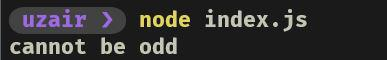When we call the doTask function with a valid value it will resolve and print the result.

Asynchronous Try/Catch: The async/await syntax supports try/catch of rejections. In other words, we can use try/catch on asynchronous promise-based APIs instead of using then and catch handler as in the next section, let’s create an async function named run and reintroduce the same try/catch pattern that was used when calling the synchronous form of doTask:

## Javascript

 `async ``function` `run() {``    ``try` `{``        ``const result = await doTask(3)``        ``console.log(``'result'``, result)``    ``} ``catch` `(err) {``        ``if` `(err ``instanceof` `TypeError) {``            ``console.error(``'wrong type'``)``        ``} ``else` `if` `(err ``instanceof` `RangeError) {``            ``console.error(``'out of range'``)``        ``} ``else` `if` `(err.code === ``'ERR_MUST_BE_EVEN'``) {``            ``console.error(``'cannot be odd'``)``        ``} ``else` `{``            ``console.error(``'Unknown error'``, err)``        ``}``    ``}``}`` ` `run()`

The only difference, other than wrapping the try/catch in an async function, is that we await doTask(3) so that the async function can handle the promise automatically. Since 3 is an odd number, the promise returned from doTask will call reject with our custom OddError and the catch block will identify the code property and then output – cannot be odd: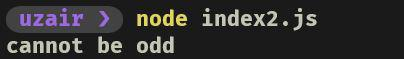This function works the same as the promise-based approach, the only difference is that it is implemented using the Try/Catch block asynchronously.

It is always good to handle the Errors at the top-most level of your code, for example- app.js in NodeJS is the entry point of the application, where all the errors should be handled.

An error can be propagated to the top-most level by re-throwing the error, consider the snippet below

## Javascript

 `const parent_function = () => {``    ``try` `{``        ``child_function()``    ``} ``catch` `(err) {``        ``console.log(``"All Errors are handled"``            ``+ ``"here in the parent function"``);``        ``console.log(`Type of Error : ``            ``\${err.name}\nError Message : \${err.message}`)``    ``}``}``const child_function = () => {``    ``try` `{``        ``throw` `new` `Error(``"Error From Child Function"``)``    ``} ``catch` `(err) {``        ``throw` `new` `Error(err.message);``    ``}``}``parent_function()`

Consider the parent_function as the entry point of the program and also observe that we handled the errors that occur in child_function inside the parent_function.

Perhaps this is confusing but, you will understand what the above snippet does once you take a look at the output: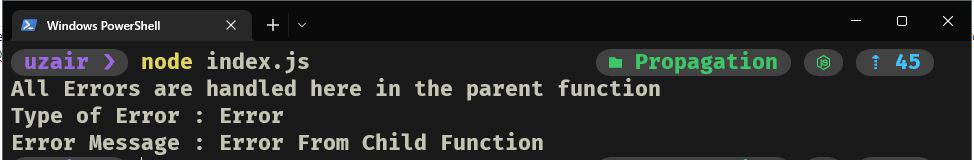My Personal Notes arrow_drop_up
Related Tutorials